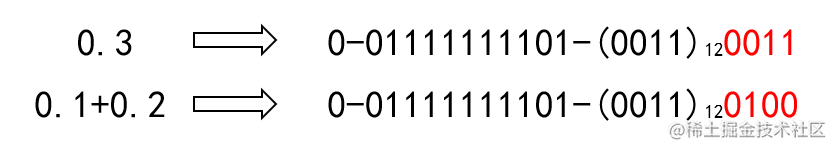# 理解JS的内存与变量存储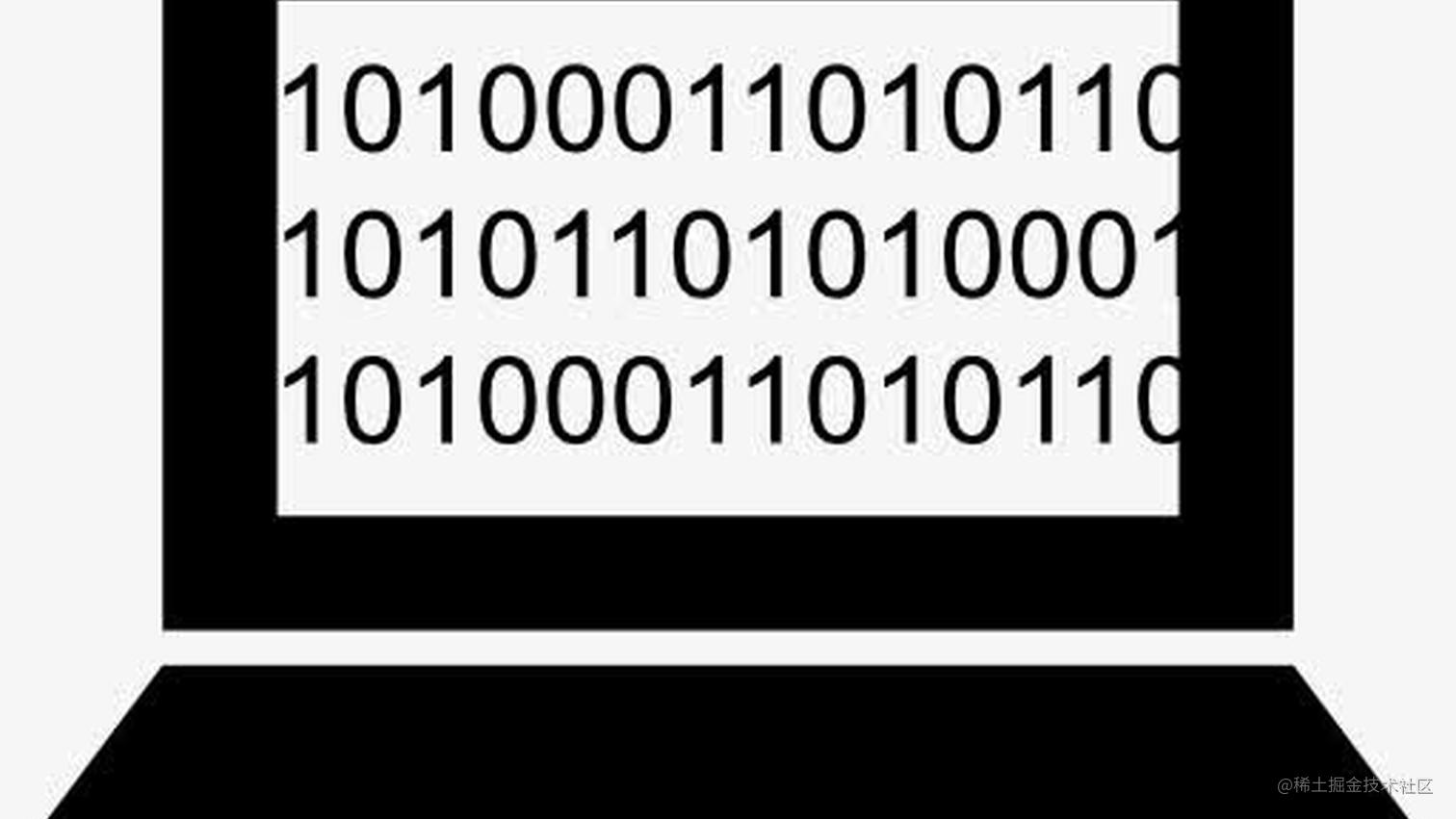## JS神奇的Number

18.9 * 100
=1889.9999999999998

0.1 + 0.2 === 0.3
// false

(function (a, b, c) {
return a + b + c === a + ( b + c )
})(0.1, 0.2, 0.3)
// false

(function (num) {
while(true) {
if (++num % 13 === 0) {
return num
}
}
})(2 ** 53)

JSON.parse('{"a":180143985094813214124}')
//{a: 180143985094813220000}

## 存储数字

106 / 2 = 53  ...... 0
53  / 2 = 26  ...... 1
26  / 2 = 13  ...... 0
13  / 2 = 6   ...... 1
6   / 2 = 3   ...... 0
3   / 2 = 1   ...... 1
1   / 2 = 0   ...... 1

0.6953125 x 2 = 1.390625  ...... 1
0.390625  x 2 = 0.78125   ...... 0
0.78125   x 2 = 1.5625    ...... 1
0.5625    x 2 = 1.125     ...... 1
0.125     x 2 = 0.25      ...... 0
0.25      x 2 = 0.5       ...... 0
0.5       x 2 = 1         ...... 1

• 使用 $8bytes(64bits)$ 存储双精度浮点数
• 存储小数用科学计数法表示的数据
• 第一位表示符号，后 $11$ 位表示指数，指数按照补位运算，即直接 $1023$ 加指数位
• 剩余 $52$ 位表示小数点后的尾数，超过 $52$ 位的部分 $0$$1$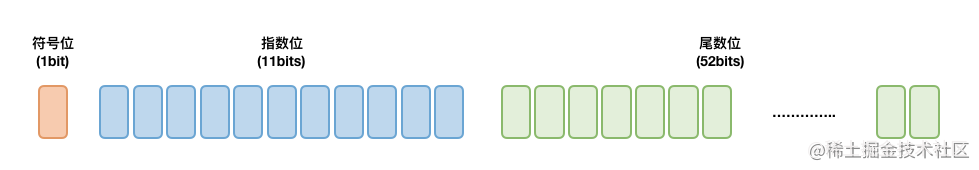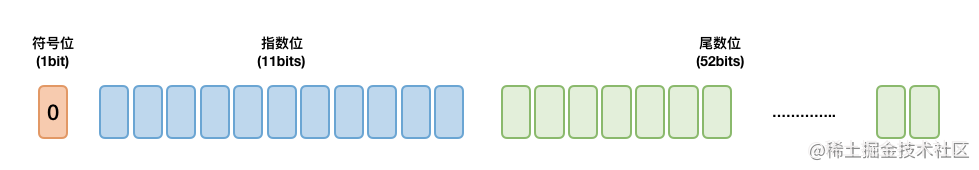• 正数的补码就是其本身
• 负数的补码是在其原码的基础上, 符号位不变, 其余各位取反, 最后+1. (即在反码的基础上+1)
[+1] = 原 = 反

[-1] = 原 = 反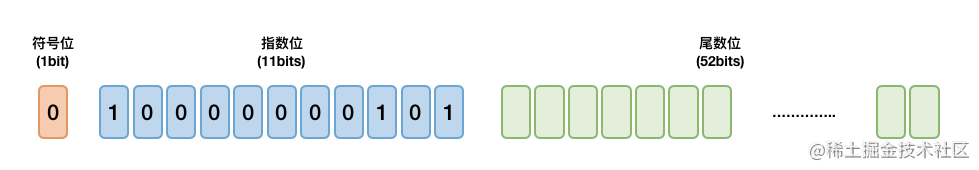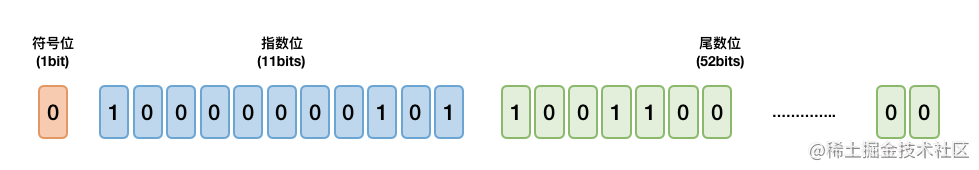## why 0.1 + 0.2 !== 0.3?

0.1 x 2 = 0.2  ...... 0
0.2 x 2 = 0.4  ...... 0
0.4 x 2 = 0.8  ...... 0
0.8 x 2 = 1.6  ...... 1
0.6 x 2 = 1.2  ...... 1
0.2 x 2 = 0.4  ...... 0
0.4 x 2 = 0.8  ...... 0
0.8 x 2 = 1.6  ...... 1
0.6 x 2 = 1.2  ...... 1

$0.1 \rightarrow 0.00011(0011)_\infty$

0.2 x 2 = 0.4  ...... 0
0.4 x 2 = 0.8  ...... 0
0.8 x 2 = 1.6  ...... 1
0.6 x 2 = 1.2  ...... 1
0.2 x 2 = 0.4  ...... 0
0.4 x 2 = 0.8  ...... 0
0.8 x 2 = 1.6  ...... 1
0.6 x 2 = 1.2  ...... 1
0.2 x 2 = 0.4  ...... 0

$0.2 \rightarrow 0.00110(0110)_\infty$

0.3 x 2 = 0.6  ...... 0
0.6 x 2 = 1.2  ...... 1
0.2 x 2 = 0.4  ...... 0
0.4 x 2 = 0.8  ...... 0
0.8 x 2 = 1.6  ...... 1
0.6 x 2 = 1.2  ...... 1
0.2 x 2 = 0.4  ...... 0
0.4 x 2 = 0.8  ...... 0
0.8 x 2 = 1.6  ...... 1

$0.3 \rightarrow 0.01001(1001)_\infty$

$0.1 \rightarrow 0.00011(0011)_\infty \rightarrow 1.(1001)_\infty*2^{-4}$

$0.2 \rightarrow 0.00110(0110)_\infty \rightarrow 1.(1001)_\infty*2^{-3}$

$0.3 \rightarrow 0.01001(1001)_\infty \rightarrow 1.(0011)_\infty*2^{-2}$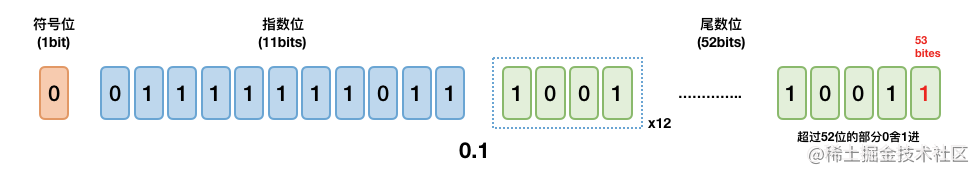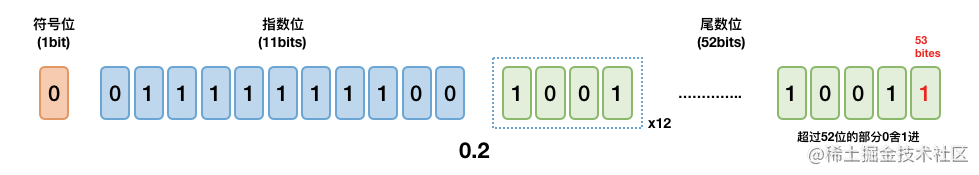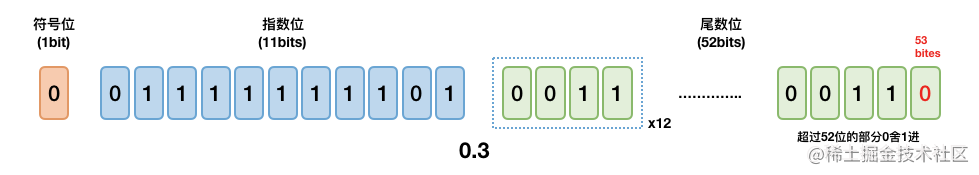$0.1 \rightarrow 0-01111111011-(1001)_{12}1010$

$0.2 \rightarrow 0-01111111100-(1001)_{12}1010$

$0.3 \rightarrow 0-01111111101-(0011)_{12}0011$